Theory and Modern Applications

# Existence results for nonlocal boundary value problems of nonlinear fractional q-difference equations

## Abstract

In this paper, we study a nonlinear fractional q-difference equation with nonlocal boundary conditions. The existence of solutions for the problem is shown by applying some well-known tools of fixed-point theory such as Banach’s contraction principle, Krasnoselskii’s fixed-point theorem, and the Leray-Schauder nonlinear alternative. Some illustrating examples are also discussed.

MSC:34A08, 39A05, 39A12, 39A13.

## 1 Introduction

In recent years, the topic of fractional differential equations has gained considerable attention and has evolved as an interesting and popular field of research. It is mainly due to the fact that several times the tools of fractional calculus are found to be more practical and effective than the corresponding ones of classical calculus in the mathematical modeling of dynamical systems associated with phenomena such as fractals and chaos. In fact, fractional calculus has numerous applications in various disciplines of science and engineering such as mechanics, electricity, chemistry, biology, economics, control theory, signal and image processing, polymer rheology, regular variation in thermodynamics, biophysics, blood flow phenomena, aerodynamics, electrodynamics of complex medium, viscoelasticity and damping, control theory, wave propagation, percolation, identification, fitting of experimental data, etc. . The development of fractional calculus ranges from the theoretical aspects of existence and uniqueness of solutions to the analytic and numerical methods for finding solutions. For some recent work on fractional differential equations, we refer to  and the references therein.

The pioneer work on q-difference calculus or quantum calculus dates back to Jackson’s papers [14, 15], while a systematic treatment of the subject can be found in [16, 17]. For some recent existence results on q-difference equations, see  and the references therein.

There has also been a growing interest on the subject of discrete fractional equations on the time scale $\mathbb{Z}$. Some interesting results on the topic can be found in a series of papers .

Fractional q-difference equations have recently attracted the attention of several researchers. For some earlier work on the topic, we refer to  and , whereas some recent work on the existence theory of fractional q-difference equations can be found in . However, the study of boundary value problems of fractional q-difference equations is at its infancy and much of the work on the topic is yet to be done.

In this paper, we discuss the existence and uniqueness of solutions for the nonlocal boundary value problem of fractional q-difference equations given by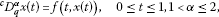(1.1)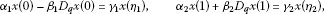(1.2)

where $f\in C\left(\left[0,1\right]×\mathbb{R},\mathbb{R}\right)$, ${}^{c}D_{q}^{\alpha }$ is the fractional q-derivative of the Caputo type, and ${\alpha }_{i},{\beta }_{i},{\gamma }_{i},{\eta }_{i}\in \mathbb{R}$ ($i=1,2$).

## 2 Preliminaries on fractional q-calculus

In this section, we cite some definitions and fundamental results of the q-calculus as well as of the fractional q-calculus [37, 38]. We also give a lemma that will be used in obtaining the main results of the paper.

Let $q\in \left(0,1\right)$ and define

${\left[a\right]}_{q}=\frac{{q}^{a}-1}{q-1},\phantom{\rule{1em}{0ex}}a\in \mathbb{R}.$

The q analogue of the power ${\left(a-b\right)}^{n}$ is

${\left(a-b\right)}^{\left(0\right)}=1,\phantom{\rule{2em}{0ex}}{\left(a-b\right)}^{\left(n\right)}=\prod _{k=0}^{n-1}\left(a-b{q}^{k}\right),\phantom{\rule{1em}{0ex}}a,b\in \mathbb{R},n\in \mathbb{N}.$

The q-gamma function is defined by

${\mathrm{\Gamma }}_{q}\left(x\right)=\frac{{\left(1-q\right)}^{\left(x-1\right)}}{{\left(1-q\right)}^{x-1}},\phantom{\rule{1em}{0ex}}x\in \mathbb{R}\setminus \left\{0,-1,-2,\dots \right\},0

and satisfies ${\mathrm{\Gamma }}_{q}\left(x+1\right)={\left[x\right]}_{q}{\mathrm{\Gamma }}_{q}\left(x\right)$ (see, ).

For $0, we define the q-derivative of a real valued function f as

${D}_{q}f\left(t\right)=\frac{f\left(t\right)-f\left(qt\right)}{\left(1-q\right)t},\phantom{\rule{1em}{0ex}}t\in {I}_{q}-\left\{0\right\},\phantom{\rule{2em}{0ex}}{D}_{q}f\left(0\right)=\underset{t\to 0}{lim}{D}_{q}f\left(t\right).$

The higher order q-derivatives are given by

${D}_{q}^{0}f\left(t\right)=f\left(t\right),\phantom{\rule{2em}{0ex}}{D}_{q}^{n}f\left(t\right)={D}_{q}{D}_{q}^{n-1}f\left(t\right),\phantom{\rule{1em}{0ex}}n\in \mathbb{N}.$

For $x\ge 0$, we set ${J}_{x}=\left\{x{q}^{n}:n\in \mathbb{N}\cup \left\{0\right\}\right\}\cup \left\{0\right\}$ and define the definite q-integral of a function $f:{J}_{x}\to \mathbb{R}$ by

${I}_{q}f\left(x\right)={\int }_{0}^{x}f\left(s\right)\phantom{\rule{0.2em}{0ex}}{d}_{q}s=\sum _{n=0}^{\mathrm{\infty }}x\left(1-q\right){q}^{n}f\left(x{q}^{n}\right)$

provided that the series converges.

For $a,b\in {J}_{x}$, we set

${\int }_{a}^{b}f\left(s\right)\phantom{\rule{0.2em}{0ex}}{d}_{q}s={I}_{q}f\left(b\right)-{I}_{q}f\left(a\right)=\left(1-q\right)\sum _{n=0}^{\mathrm{\infty }}{q}^{n}\left[bf\left(b{q}^{n}\right)-af\left(a{q}^{n}\right)\right],$

provided that the series exist. Throughout the paper, we will assume that the series in the q-integrals converge.

Note that for $a,b\in {J}_{x}$, we have $a=x{q}^{{n}_{1}}$, $b=x{q}^{{n}_{2}}$ for some ${n}_{1},{n}_{2}\in \mathbb{N}$, thus the definite integral ${\int }_{a}^{b}f\left(s\right)\phantom{\rule{0.2em}{0ex}}{d}_{q}s$ is just a finite sum, so no question about convergence is raised.

We note that

${D}_{q}{I}_{q}f\left(x\right)=f\left(x\right),$

while if f is continuous at $x=0$, then

${I}_{q}{D}_{q}f\left(x\right)=f\left(x\right)-f\left(0\right).$

For more details of the basic material on q-calculus, see the book .

Definition 2.1 ()

Let $\alpha \ge 0$ and f be a function defined on $\left[0,1\right]$. The fractional q-integral of the Riemann-Liouville type is $\left({I}_{q}^{0}f\right)\left(t\right)=f\left(t\right)$ and

$\left({I}_{q}^{\alpha }f\right)\left(t\right)={\int }_{0}^{t}\frac{{\left(t-qs\right)}^{\left(\alpha -1\right)}}{{\mathrm{\Gamma }}_{q}\left(\alpha \right)}f\left(s\right)\phantom{\rule{0.2em}{0ex}}{d}_{q}\left(s\right),\phantom{\rule{1em}{0ex}}\alpha >0,t\in \left[0,1\right].$

Definition 2.2 ()

The fractional q-derivative of the Riemann-Liouville type of order $\alpha \ge 0$ is defined by $\left({D}_{q}^{0}f\right)\left(t\right)=f\left(t\right)$ and

$\left({D}_{q}^{\alpha }f\right)\left(t\right)=\left({D}_{q}^{\left[\alpha \right]}{I}_{q}^{\left[\alpha \right]-\alpha }f\right)\left(t\right),\phantom{\rule{1em}{0ex}}\alpha >0,$

where $\left[\alpha \right]$ is the smallest integer greater than or equal to α.

Definition 2.3 ()

The fractional q-derivative of the Caputo type of order $\alpha \ge 0$ is defined by

${\left(}^{c}{D}_{q}^{\alpha }f\right)\left(t\right)=\left({I}_{q}^{\left[\alpha \right]-\alpha }{D}_{q}^{\left[\alpha \right]}f\right)\left(t\right),\phantom{\rule{1em}{0ex}}\alpha >0,$

where $\left[\alpha \right]$ is the smallest integer greater than or equal to α.

Lemma 2.4 Let $\alpha ,\beta \ge 0$ and let f be a function defined on $\left[0,1\right]$. Then the next formulas hold:

1. (i)

$\left({I}_{q}^{\beta }{I}_{q}^{\alpha }f\right)\left(t\right)=\left({I}_{q}^{\alpha +\beta }f\right)\left(t\right)$,

2. (ii)

$\left({D}_{q}^{\alpha }{I}_{q}^{\alpha }f\right)\left(t\right)=f\left(t\right)$.

Lemma 2.5 ()

Let $\alpha \ge 0$ and $n\in \mathbb{N}$. Then the following equality holds:

$\left({I}_{q}^{\alpha }{D}_{q}^{n}f\right)\left(t\right)={D}_{q}^{n}{I}_{q}^{\alpha }f\left(t\right)-\sum _{k=0}^{\left[\alpha \right]-1}\frac{{t}^{\alpha -n+k}}{{\mathrm{\Gamma }}_{q}\left(\alpha -n+k\right)}\left({D}_{q}^{k}f\right)\left(0\right).$

Lemma 2.6 ()

Let $\alpha >0$. Then the following equality holds:

$\left({{I}_{q}^{\alpha }}^{c}{D}_{q}^{\alpha }f\right)\left(t\right)=f\left(t\right)-\sum _{k=0}^{\left[\alpha \right]-1}\frac{{t}^{k}}{{\mathrm{\Gamma }}_{q}\left(k+1\right)}\left({D}_{q}^{k}f\right)\left(0\right).$

Lemma 2.7 ()

For $\alpha \in {\mathbb{R}}^{+}$, $\lambda \in \left(-1,\mathrm{\infty }\right)$, the following is valid:

${I}_{q}^{\alpha }\left({\left(x-a\right)}^{\left(\lambda \right)}\right)=\frac{{\mathrm{\Gamma }}_{q}\left(\lambda +1\right)}{\mathrm{\Gamma }\left(\alpha +\lambda +1\right)}{\left(x-a\right)}^{\left(\alpha +\lambda \right)},\phantom{\rule{1em}{0ex}}0

In particular, for $\lambda =0$, $a=0$, using q-integration by parts, we have

$\begin{array}{rcl}\left({I}_{q}^{\alpha }1\right)\left(x\right)& =& \frac{1}{{\mathrm{\Gamma }}_{q}\left(\alpha \right)}{\int }_{0}^{x}{\left(x-qt\right)}^{\left(\alpha -1\right)}\phantom{\rule{0.2em}{0ex}}{d}_{q}t=\frac{1}{{\mathrm{\Gamma }}_{q}\left(\alpha \right)}{\int }_{0}^{x}\frac{{D}_{q}\left({\left(x-t\right)}^{\left(\alpha \right)}\right)}{-{\left[\alpha \right]}_{q}}\phantom{\rule{0.2em}{0ex}}{d}_{q}t\\ =& \frac{-1}{{\mathrm{\Gamma }}_{q}\left(\alpha +1\right)}{\int }_{0}^{x}{D}_{q}\left({\left(x-t\right)}^{\left(\alpha \right)}\right)\phantom{\rule{0.2em}{0ex}}{d}_{q}t=\frac{1}{{\mathrm{\Gamma }}_{q}\left(\alpha +1\right)}{x}^{\left(\alpha \right)}.\end{array}$

In order to define the solution for the problem (1.1)-(1.2), we need the following lemma.

Lemma 2.8 For a given $g\in C\left(\left[0,1\right],\mathbb{R}\right)$ the unique solution of the boundary value problem

$\left\{\begin{array}{c}{}^{c}D_{q}^{\alpha }x\left(t\right)=g\left(t\right),\phantom{\rule{1em}{0ex}}t\in \left[0,1\right],\hfill \\ {\alpha }_{1}x\left(0\right)-{\beta }_{1}{D}_{q}x\left(0\right)={\gamma }_{1}x\left({\eta }_{1}\right),\hfill \\ {\alpha }_{2}x\left(1\right)+{\beta }_{2}{D}_{q}x\left(1\right)={\gamma }_{2}x\left({\eta }_{2}\right),\hfill \end{array}$
(2.1)

is given by

$\begin{array}{rcl}x\left(t\right)& =& {\int }_{0}^{t}\frac{{\left(t-qs\right)}^{\left(\alpha -1\right)}}{{\mathrm{\Gamma }}_{q}\left(\alpha \right)}g\left(s\right)\phantom{\rule{0.2em}{0ex}}{d}_{q}s\\ +\frac{{\gamma }_{1}}{\mathrm{\Delta }}\left[\left({\alpha }_{2}-{\gamma }_{2}\right)t-\left({\alpha }_{2}+{\beta }_{2}-{\gamma }_{2}{\eta }_{2}\right)\right]{\int }_{0}^{{\eta }_{1}}\frac{{\left({\eta }_{1}-qs\right)}^{\left(\alpha -1\right)}}{{\mathrm{\Gamma }}_{q}\left(\alpha \right)}g\left(s\right)\phantom{\rule{0.2em}{0ex}}{d}_{q}s\\ -\frac{{\gamma }_{2}}{\mathrm{\Delta }}\left[\left({\alpha }_{1}-{\gamma }_{1}\right)t+\left({\beta }_{1}+{\gamma }_{1}{\eta }_{1}\right)\right]{\int }_{0}^{{\eta }_{2}}\frac{{\left({\eta }_{2}-qs\right)}^{\left(\alpha -1\right)}}{{\mathrm{\Gamma }}_{q}\left(\alpha \right)}g\left(s\right)\phantom{\rule{0.2em}{0ex}}{d}_{q}s\\ +\frac{{\alpha }_{2}}{\mathrm{\Delta }}\left[\left({\alpha }_{1}-{\gamma }_{1}\right)t+{\beta }_{1}+{\gamma }_{1}{\eta }_{1}\right]{\int }_{0}^{1}\frac{{\left(1-qs\right)}^{\left(\alpha -1\right)}}{{\mathrm{\Gamma }}_{q}\left(\alpha \right)}g\left(s\right)\phantom{\rule{0.2em}{0ex}}{d}_{q}s\\ +\frac{{\beta }_{2}}{\mathrm{\Delta }}\left[\left({\alpha }_{1}-{\gamma }_{1}\right)t+{\beta }_{1}+{\gamma }_{1}{\eta }_{1}\right]{\int }_{0}^{1}\frac{{\left(1-qs\right)}^{\left(\alpha -2\right)}}{{\mathrm{\Gamma }}_{q}\left(\alpha -1\right)}g\left(s\right)\phantom{\rule{0.2em}{0ex}}{d}_{q}s,\end{array}$
(2.2)

where

$\mathrm{\Delta }=\left({\gamma }_{2}-{\alpha }_{2}\right)\left({\beta }_{1}+{\gamma }_{1}{\eta }_{1}\right)+\left({\gamma }_{1}-{\alpha }_{1}\right)\left({\alpha }_{2}+{\beta }_{2}-{\gamma }_{2}{\eta }_{2}\right)\ne 0.$
(2.3)

Proof In view of Lemmas 2.4 and 2.6, integrating equation in (2.1), we have

$x\left(t\right)={\int }_{0}^{t}\frac{{\left(t-qs\right)}^{\left(\alpha -1\right)}}{{\mathrm{\Gamma }}_{q}\left(\alpha \right)}g\left(s\right)\phantom{\rule{0.2em}{0ex}}{d}_{q}s+{c}_{0}t+{c}_{1},\phantom{\rule{1em}{0ex}}t\in \left[0,1\right].$
(2.4)

Using the boundary conditions of (2.1) in (2.4), we have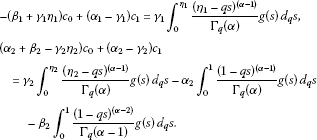Solving the above system of equations for ${c}_{0}$, ${c}_{1}$, we get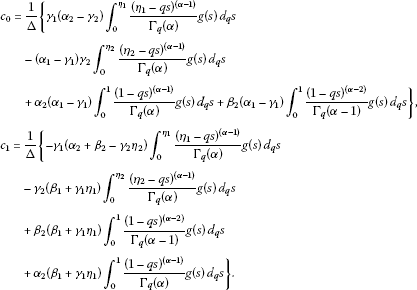Substituting the values of ${c}_{0}$, ${c}_{1}$ in (2.4), we obtain (2.2). □

In view of Lemma 2.8, we define an operator $F:C\left(\left[0,1\right],\mathbb{R}\right)\to C\left(\left[0,1\right],\mathbb{R}\right)$ as

$\begin{array}{rcl}\left(Fx\right)\left(t\right)& =& {\int }_{0}^{t}\frac{{\left(t-qs\right)}^{\left(\alpha -1\right)}}{{\mathrm{\Gamma }}_{q}\left(\alpha \right)}f\left(s,x\left(s\right)\right)\phantom{\rule{0.2em}{0ex}}{d}_{q}s\\ +\frac{{\gamma }_{1}}{\mathrm{\Delta }}\left[\left({\alpha }_{2}-{\gamma }_{2}\right)t-\left({\alpha }_{2}+{\beta }_{2}-{\gamma }_{2}{\eta }_{2}\right)\right]{\int }_{0}^{{\eta }_{1}}\frac{{\left({\eta }_{1}-qs\right)}^{\left(\alpha -1\right)}}{{\mathrm{\Gamma }}_{q}\left(\alpha \right)}f\left(s,x\left(s\right)\right)\phantom{\rule{0.2em}{0ex}}{d}_{q}s\\ -\frac{{\gamma }_{2}}{\mathrm{\Delta }}\left[\left({\alpha }_{1}-{\gamma }_{1}\right)t+\left({\beta }_{1}+{\gamma }_{1}{\eta }_{1}\right)\right]{\int }_{0}^{{\eta }_{2}}\frac{{\left({\eta }_{2}-qs\right)}^{\left(\alpha -1\right)}}{{\mathrm{\Gamma }}_{q}\left(\alpha \right)}f\left(s,x\left(s\right)\right)\phantom{\rule{0.2em}{0ex}}{d}_{q}s\\ +\frac{{\alpha }_{2}}{\mathrm{\Delta }}\left[\left({\alpha }_{1}-{\gamma }_{1}\right)t+\left({\beta }_{1}+{\gamma }_{1}{\eta }_{1}\right)\right]{\int }_{0}^{1}\frac{{\left(1-qs\right)}^{\left(\alpha -1\right)}}{{\mathrm{\Gamma }}_{q}\left(\alpha \right)}f\left(s,x\left(s\right)\right)\phantom{\rule{0.2em}{0ex}}{d}_{q}s\\ +\frac{{\beta }_{2}}{\mathrm{\Delta }}\left[\left({\alpha }_{1}-{\gamma }_{1}\right)t+\left({\beta }_{1}+{\gamma }_{1}{\eta }_{1}\right)\right]{\int }_{0}^{1}\frac{{\left(1-qs\right)}^{\left(\alpha -2\right)}}{{\mathrm{\Gamma }}_{q}\left(\alpha -1\right)}f\left(s,x\left(s\right)\right)\phantom{\rule{0.2em}{0ex}}{d}_{q}s.\end{array}$
(2.5)

Observe that problem (1.1)-(1.2) has a solution if the operator equation $Fx=x$ has a fixed point, where F is given by (2.5).

## 3 Main results

Let $\mathcal{C}:=C\left(\left[0,1\right],\mathbb{R}\right)$ denote the Banach space of all continuous functions from $\left[0,1\right]\to \mathbb{R}$ endowed with the norm defined by $\parallel x\parallel =sup\left\{|x\left(t\right)|:t\in \left[0,1\right]\right\}$.

For the sake of convenience, we set

$k:=\left(1+|{\alpha }_{2}|{\delta }_{2}\right)\left({I}_{q}^{\alpha }L\right)\left(1\right)+|{\gamma }_{1}|{\delta }_{1}\left({I}_{q}^{\alpha }L\right)\left({\eta }_{1}\right)+|{\gamma }_{2}|{\delta }_{2}\left({I}_{q}^{\alpha }L\right)\left({\eta }_{2}\right)+|{\beta }_{2}|{\delta }_{2}\left({I}_{q}^{\alpha -1}L\right)\left(1\right),$
(3.1)

where

${\delta }_{1}:=\frac{|{\alpha }_{2}-{\gamma }_{2}|+|{\alpha }_{2}+{\beta }_{2}-{\gamma }_{2}{\eta }_{2}|}{|\mathrm{\Delta }|},$
(3.2)

and

${\delta }_{2}:=\frac{|{\alpha }_{1}-{\gamma }_{1}|+|{\beta }_{1}+{\gamma }_{1}{\eta }_{1}|}{|\mathrm{\Delta }|}.$
(3.3)

Theorem 3.1 Assume that $f:\left[0,1\right]×\mathbb{R}\to \mathbb{R}$ is continuous and that there exists a q-integrable function $L:\left[0,1\right]\to \mathbb{R}$ such that

(A1) $|f\left(t,x\right)-f\left(t,y\right)|\le L\left(t\right)|x-y|$, $t\in \left[0,1\right]$, $x,y\in \mathbb{R}$.

Then the boundary value problem (1.1)-(1.2) has a unique solution provided

$k<1,$
(3.4)

where k is given by (3.1).

Proof Let us fix ${sup}_{t\in \left[0,1\right]}|f\left(t,0\right)|=M$ and choose

$\rho \ge \frac{MA}{1-k},$

where

$A=\frac{1}{{\mathrm{\Gamma }}_{q}\left(\alpha +1\right)}\left(1+|{\gamma }_{1}|{\delta }_{1}{\eta }_{1}^{\left(\alpha -1\right)}+|{\gamma }_{2}|{\delta }_{2}{\eta }_{2}^{\left(\alpha -1\right)}+|{\alpha }_{2}|{\delta }_{2}\right)+\frac{|{\beta }_{2}|{\delta }_{2}}{{\mathrm{\Gamma }}_{q}\left(\alpha \right)}.$
(3.5)

We define ${B}_{\rho }=\left\{x\in \mathcal{C}:\parallel x\parallel \le \rho \right\}$ and show that $F{B}_{\rho }\subset {B}_{\rho }$, where F is defined by (2.5). For $x\in {B}_{\rho }$, observe that

$|f\left(t,x\left(t\right)\right)|\le |f\left(t,x\left(t\right)\right)-f\left(t,0\right)|+|f\left(t,0\right)|\le L\left(t\right)|x\left(t\right)|+|f\left(t,0\right)|\le L\left(t\right)\rho +M.$

Then $x\in {B}_{\rho }$, $t\in \left[0,1\right]$, we have

$\begin{array}{rcl}|\left(Fx\right)\left(t\right)|& \le & {\int }_{0}^{t}\frac{{\left(t-qs\right)}^{\left(\alpha -1\right)}}{{\mathrm{\Gamma }}_{q}\left(\alpha \right)}\left[L\left(s\right)\rho +M\right]\phantom{\rule{0.2em}{0ex}}{d}_{q}s\\ +|{\gamma }_{1}|{\delta }_{1}{\int }_{0}^{{\eta }_{1}}\frac{{\left({\eta }_{1}-qs\right)}^{\left(\alpha -1\right)}}{{\mathrm{\Gamma }}_{q}\left(\alpha \right)}\left[L\left(s\right)\rho +M\right]\phantom{\rule{0.2em}{0ex}}{d}_{q}s\\ +|{\gamma }_{2}|{\delta }_{2}{\int }_{0}^{{\eta }_{2}}\frac{{\left({\eta }_{2}-qs\right)}^{\left(\alpha -1\right)}}{{\mathrm{\Gamma }}_{q}\left(\alpha \right)}\left[L\left(s\right)\rho +M\right]\phantom{\rule{0.2em}{0ex}}{d}_{q}s\\ +|{\alpha }_{2}|{\delta }_{2}{\int }_{0}^{1}\frac{{\left(1-qs\right)}^{\left(\alpha -1\right)}}{{\mathrm{\Gamma }}_{q}\left(\alpha \right)}\left[L\left(s\right)\rho +M\right]\phantom{\rule{0.2em}{0ex}}{d}_{q}s\\ +|{\beta }_{2}|{\delta }_{2}{\int }_{0}^{1}\frac{{\left(1-qs\right)}^{\left(\alpha -2\right)}}{{\mathrm{\Gamma }}_{q}\left(\alpha -1\right)}\left[L\left(s\right)\rho +M\right]\phantom{\rule{0.2em}{0ex}}{d}_{q}s\\ \le & M\left\{{\int }_{0}^{1}\frac{{\left(1-qs\right)}^{\left(\alpha -1\right)}}{{\mathrm{\Gamma }}_{q}\left(\alpha \right)}\phantom{\rule{0.2em}{0ex}}{d}_{q}s+|{\gamma }_{1}|{\delta }_{1}{\int }_{0}^{{\eta }_{1}}\frac{{\left({\eta }_{1}-qs\right)}^{\left(\alpha -1\right)}}{{\mathrm{\Gamma }}_{q}\left(\alpha \right)}\phantom{\rule{0.2em}{0ex}}{d}_{q}s\\ +|{\gamma }_{2}|{\delta }_{2}{\int }_{0}^{{\eta }_{2}}\frac{{\left({\eta }_{2}-qs\right)}^{\left(\alpha -1\right)}}{{\mathrm{\Gamma }}_{q}\left(\alpha \right)}\phantom{\rule{0.2em}{0ex}}{d}_{q}s\\ +|{\alpha }_{2}|{\delta }_{2}{\int }_{0}^{1}\frac{{\left(1-qs\right)}^{\left(\alpha -1\right)}}{{\mathrm{\Gamma }}_{q}\left(\alpha \right)}\phantom{\rule{0.2em}{0ex}}{d}_{q}s+|{\beta }_{2}|{\delta }_{2}{\int }_{0}^{1}\frac{{\left(1-qs\right)}^{\left(\alpha -2\right)}}{{\mathrm{\Gamma }}_{q}\left(\alpha -1\right)}\phantom{\rule{0.2em}{0ex}}{d}_{q}s\right\}\\ +\rho \left\{{\int }_{0}^{1}\frac{{\left(1-qs\right)}^{\left(\alpha -1\right)}}{{\mathrm{\Gamma }}_{q}\left(\alpha \right)}L\left(s\right)\phantom{\rule{0.2em}{0ex}}{d}_{q}s+|{\gamma }_{1}|{\delta }_{1}{\int }_{0}^{{\eta }_{1}}\frac{{\left({\eta }_{1}-qs\right)}^{\left(\alpha -1\right)}}{{\mathrm{\Gamma }}_{q}\left(\alpha \right)}L\left(s\right)\phantom{\rule{0.2em}{0ex}}{d}_{q}s\\ +|{\gamma }_{2}|{\delta }_{2}{\int }_{0}^{{\eta }_{2}}\frac{{\left({\eta }_{2}-qs\right)}^{\left(\alpha -1\right)}}{{\mathrm{\Gamma }}_{q}\left(\alpha \right)}L\left(s\right)\phantom{\rule{0.2em}{0ex}}{d}_{q}s\\ +|{\alpha }_{2}|{\delta }_{2}{\int }_{0}^{1}\frac{{\left(1-qs\right)}^{\left(\alpha -1\right)}}{{\mathrm{\Gamma }}_{q}\left(\alpha \right)}L\left(s\right)\phantom{\rule{0.2em}{0ex}}{d}_{q}s+|{\beta }_{2}|{\delta }_{2}{\int }_{0}^{1}\frac{{\left(1-qs\right)}^{\left(\alpha -2\right)}}{{\mathrm{\Gamma }}_{q}\left(\alpha -1\right)}L\left(s\right)\phantom{\rule{0.2em}{0ex}}{d}_{q}s\right\}\\ \le & M\left\{\frac{1}{{\mathrm{\Gamma }}_{q}\left(\alpha +1\right)}\left(1+|{\gamma }_{1}|{\delta }_{1}{\eta }_{1}^{\left(\alpha -1\right)}+|{\gamma }_{2}|{\delta }_{2}{\eta }_{2}^{\left(\alpha -1\right)}+|{\alpha }_{2}|{\delta }_{2}\right)+\frac{|{\beta }_{2}|{\delta }_{2}}{{\mathrm{\Gamma }}_{q}\left(\alpha \right)}\right\}\\ +\rho \left\{{\int }_{0}^{1}\frac{{\left(1-qs\right)}^{\left(\alpha -1\right)}}{{\mathrm{\Gamma }}_{q}\left(\alpha \right)}L\left(s\right)\phantom{\rule{0.2em}{0ex}}{d}_{q}s+|{\gamma }_{1}|{\delta }_{1}{\int }_{0}^{{\eta }_{1}}\frac{{\left({\eta }_{1}-qs\right)}^{\left(\alpha -1\right)}}{{\mathrm{\Gamma }}_{q}\left(\alpha \right)}L\left(s\right)\phantom{\rule{0.2em}{0ex}}{d}_{q}s\\ +|{\gamma }_{2}|{\delta }_{2}{\int }_{0}^{{\eta }_{2}}\frac{{\left({\eta }_{2}-qs\right)}^{\left(\alpha -1\right)}}{{\mathrm{\Gamma }}_{q}\left(\alpha \right)}L\left(s\right)\phantom{\rule{0.2em}{0ex}}{d}_{q}s+|{\alpha }_{2}|{\delta }_{2}{\int }_{0}^{1}\frac{{\left(1-qs\right)}^{\left(\alpha -1\right)}}{{\mathrm{\Gamma }}_{q}\left(\alpha \right)}L\left(s\right)\phantom{\rule{0.2em}{0ex}}{d}_{q}s\\ +|{\beta }_{2}|{\delta }_{2}{\int }_{0}^{1}\frac{{\left(1-qs\right)}^{\left(\alpha -2\right)}}{{\mathrm{\Gamma }}_{q}\left(\alpha -1\right)}L\left(s\right)\phantom{\rule{0.2em}{0ex}}{d}_{q}s\right\},\end{array}$

which, in view of (3.1) and (3.5), implies that

$\parallel Fx\parallel \le MA+\rho k\le \rho .$

This shows that $F{B}_{\rho }\subset {B}_{\rho }$.

Now, for $x,y\in \mathcal{C}$, we obtain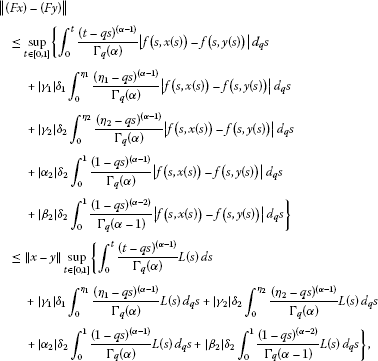which, in view of (3.1), yields

$\parallel \left(Fx\right)\left(t\right)-\left(Fy\right)\left(t\right)\parallel \le k\parallel x-y\parallel .$

Since $k\in \left(0,1\right)$ by assumption (3.4), therefore, F is a contraction. Hence, it follows by Banach’s contraction principle that the problem (1.1)-(1.2) has a unique solution. □

In case $L\left(t\right)=L$ (L is a constant), the condition (3.4) becomes $LA<1$ and Theorem 3.1 takes the form of the following result.

Corollary 3.2 Assume that $f:\left[0,1\right]×\mathbb{R}\to \mathbb{R}$ is a continuous function and that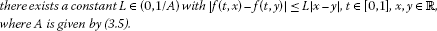Then the boundary value problem (1.1)-(1.2) has a unique solution.

Our next existence results is based on Krasnoselskii’s fixed-point theorem .

Lemma 3.3 (Krasnoselskii)

Let M be a closed, bounded, convex, and nonempty subset of a Banach space X. Let A, B be two operators such that:

1. (i)

$Ax+By\in M$ whenever $x,y\in M$;

2. (ii)

A is compact and continuous;

3. (iii)

B is a contraction mapping.

Then there exists $z\in M$ such that $z=Az+Bz$.

Theorem 3.4 Let $f:\left[0,1\right]×\mathbb{R}\to \mathbb{R}$ be a continuous function satisfying (A1). In addition, we assume that

(A2) there exists a function $\mu \in C\left(\left[0,1\right],{\mathbb{R}}^{+}\right)$ and a nondecreasing function $\varphi \in C\left(\left[0,1\right],{\mathbb{R}}^{+}\right)$ with

$|f\left(t,x\right)|\le \mu \left(t\right)\varphi \left(|x|\right),\phantom{\rule{1em}{0ex}}\left(t,x\right)\in \left[0,1\right]×\mathbb{R};$

(A3) there exists a constant $\overline{r}$ with

$\begin{array}{rcl}\overline{r}& \ge & \left[\frac{1}{{\mathrm{\Gamma }}_{q}\left(\alpha +1\right)}+|{\gamma }_{1}|{\delta }_{1}\frac{{\eta }_{1}^{\left(\alpha -1\right)}}{{\mathrm{\Gamma }}_{q}\left(\alpha +1\right)}+|{\gamma }_{2}|{\delta }_{2}\frac{{\eta }_{2}^{\left(\alpha -1\right)}}{{\mathrm{\Gamma }}_{q}\left(\alpha +1\right)}\\ +\frac{|{\alpha }_{2}|{\delta }_{2}}{{\mathrm{\Gamma }}_{q}\left(\alpha +1\right)}+\frac{|{\beta }_{2}|{\delta }_{2}}{{\mathrm{\Gamma }}_{q}\left(\alpha \right)}\right]\varphi \left(\overline{r}\right)\parallel \mu \parallel ,\end{array}$
(3.6)

where $\parallel \mu \parallel ={sup}_{t\in \left[0,T\right]}|\mu \left(t\right)|$.

If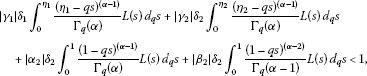(3.7)

then the boundary value problem (1.1)-(1.2) has at least one solution on $\left[0,1\right]$.

Proof Consider the set ${B}_{\overline{r}}=\left\{x\in \mathcal{C}:\parallel x\parallel \le \overline{r}\right\}$, where $\overline{r}$ is given in (3.6) and define the operators $\mathcal{P}$ and $\mathcal{Q}$ on ${B}_{\overline{r}}$ as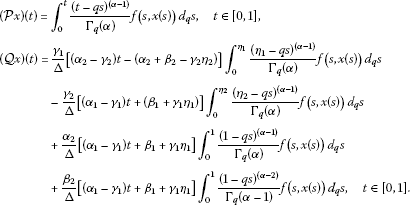For $x,y\in {B}_{\overline{r}}$, we find that

$\begin{array}{rcl}|\left(\mathcal{P}x+\mathcal{Q}y\right)\left(t\right)|& \le & {\int }_{0}^{t}\frac{{\left(t-qs\right)}^{\left(\alpha -1\right)}}{{\mathrm{\Gamma }}_{q}\left(\alpha \right)}\mu \left(s\right)\varphi \left(|x\left(s\right)|\right)\phantom{\rule{0.2em}{0ex}}{d}_{q}s\\ +|{\gamma }_{1}|{\delta }_{1}{\int }_{0}^{{\eta }_{1}}\frac{{\left({\eta }_{1}-qs\right)}^{\left(\alpha -1\right)}}{{\mathrm{\Gamma }}_{q}\left(\alpha \right)}\mu \left(s\right)\varphi \left(|x\left(s\right)|\right)\phantom{\rule{0.2em}{0ex}}{d}_{q}s\\ +{\gamma }_{2}{\delta }_{2}{\int }_{0}^{{\eta }_{2}}\frac{{\left({\eta }_{2}-qs\right)}^{\left(\alpha -1\right)}}{{\mathrm{\Gamma }}_{q}\left(\alpha \right)}\mu \left(s\right)\varphi \left(|x\left(s\right)|\right)\phantom{\rule{0.2em}{0ex}}{d}_{q}s\\ +|{\alpha }_{2}|{\delta }_{2}{\int }_{0}^{1}\frac{{\left(1-qs\right)}^{\left(\alpha -1\right)}}{{\mathrm{\Gamma }}_{q}\left(\alpha \right)}\mu \left(s\right)\varphi \left(|x\left(s\right)|\right)\phantom{\rule{0.2em}{0ex}}{d}_{q}s\\ +|{\beta }_{2}|{\delta }_{2}{\int }_{0}^{1}\frac{{\left(1-qs\right)}^{\left(\alpha -2\right)}}{{\mathrm{\Gamma }}_{q}\left(\alpha -1\right)}\mu \left(s\right)\varphi \left(|x\left(s\right)|\right)\phantom{\rule{0.2em}{0ex}}{d}_{q}s\\ \le & \varphi \left(\overline{r}\right)\parallel \mu \parallel \left\{\frac{1}{{\mathrm{\Gamma }}_{q}\left(\alpha +1\right)}+|{\gamma }_{1}|{\delta }_{1}\frac{{\eta }_{1}^{\left(\alpha -1\right)}}{{\mathrm{\Gamma }}_{q}\left(\alpha +1\right)}+|{\gamma }_{2}|{\delta }_{2}\frac{{\eta }_{2}^{\left(\alpha -1\right)}}{{\mathrm{\Gamma }}_{q}\left(\alpha +1\right)}\\ +|{\alpha }_{2}|{\delta }_{2}\frac{1}{{\mathrm{\Gamma }}_{q}\left(\alpha +1\right)}+|{\beta }_{2}|{\delta }_{2}\frac{1}{{\mathrm{\Gamma }}_{q}\left(\alpha \right)}\right\}\\ \le & \overline{r}.\end{array}$

Thus, $\mathcal{P}x+\mathcal{Q}y\in {B}_{\overline{r}}$. From (A1) and (3.7) it follows that $\mathcal{Q}$ is a contraction mapping. Continuity of f implies that the operator $\mathcal{P}$ is continuous. Also, $\mathcal{P}$ is uniformly bounded on ${B}_{\overline{r}}$ as

$\parallel \mathcal{P}x\parallel \le \frac{\varphi \left(\overline{r}\right)}{{\mathrm{\Gamma }}_{q}\left(\alpha +1\right)}\parallel \mu \parallel .$

Now, for any $x\in {B}_{\overline{r}}$, and ${t}_{1},{t}_{2}\in \left[0,1\right]$ with ${t}_{1}<{t}_{2}$, we have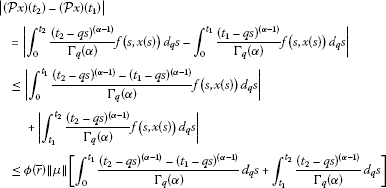which is independent of x and tends to zero as ${t}_{2}\to {t}_{1}$. Thus, $\mathcal{P}$ is equicontinuous. So $\mathcal{P}$ is relatively compact on ${B}_{\overline{r}}$. Hence, by the Arzelá-Ascoli theorem, $\mathcal{P}$ is compact on ${B}_{\overline{r}}$. Thus, all the assumptions of Lemma 3.3 are satisfied. So the conclusion of Lemma 3.3 implies that the boundary value problem (1.1)-(1.2) has at least one solution on $\left[0,1\right]$. □

In the special case when $\varphi \left(u\right)\equiv 1$, we see that there always exists a positive r so that (3.6) holds true, thus we have the following corollary.

Corollary 3.5 Let $f:\left[0,1\right]×\mathbb{R}\to \mathbb{R}$ be a continuous function satisfying (A1). In addition, we assume that

$|f\left(t,x\right)|\le \mu \left(t\right),\phantom{\rule{1em}{0ex}}\mathrm{\forall }\left(t,x\right)\in \left[0,1\right]×\mathbb{R},\phantom{\rule{0.25em}{0ex}}\mathit{\text{and}}\phantom{\rule{0.25em}{0ex}}\mu \in C\left(\left[0,1\right],{\mathbb{R}}^{+}\right).$

If (3.7) holds, then the boundary value problem (1.1)-(1.2) has at least one solution on $\left[0,1\right]$.

The next existence result is based on Leray-Schauder nonlinear alternative.

Lemma 3.6 (Nonlinear alternative for single valued maps )

Let E be a Banach space, C a closed, convex subset of E, U an open subset of C with $0\in U$. Suppose that $F:\overline{U}\to C$ is a continuous, compact (that is, $F\left(\overline{U}\right)$ is a relatively compact subset of C) map. Then either

1. (i)

F has a fixed point in $\overline{U}$, or

2. (ii)

there is a $u\in \partial U$ (the boundary of U in C) and $\lambda \in \left(0,1\right)$ with $u=\lambda F\left(u\right)$.

Theorem 3.7 Let $f:\left[0,1\right]×\mathbb{R}\to \mathbb{R}$ be a continuous function. Assume that:

(A4) there exist functions ${p}_{1},{p}_{2}\in {L}^{1}\left(\left[0,1\right],{\mathbb{R}}^{+}\right)$, and a nondecreasing function $\psi :{\mathbb{R}}^{+}\to {\mathbb{R}}^{+}$ such that $|f\left(t,x\right)|\le {p}_{1}\left(t\right)\psi \left(|x|\right)+{p}_{2}\left(t\right),\phantom{\rule{0.25em}{0ex}}\mathit{\text{for}}\phantom{\rule{0.25em}{0ex}}\left(t,x\right)\in \left[0,1\right]×\mathbb{R}$;

(A5) there exists a number $M>0$ such that

$\frac{M}{\psi \left(M\right){\omega }_{1}+{\omega }_{2}}>1,$
(3.8)

where

$\begin{array}{rcl}{\omega }_{i}& :=& \left(1+|{\alpha }_{2}|{\delta }_{2}\right)\left({I}_{q}^{\alpha }{p}_{i}\right)\left(1\right)+|{\gamma }_{1}|{\delta }_{1}\left({I}_{q}^{\alpha }{p}_{i}\right)\left({\eta }_{1}\right)+|{\gamma }_{2}|{\delta }_{2}\left({I}_{q}^{\alpha }{p}_{i}\right)\left({\eta }_{2}\right)\\ +|{\beta }_{2}|{\delta }_{2}\left({I}_{q}^{\alpha -1}{p}_{i}\right)\left(1\right),\phantom{\rule{1em}{0ex}}i=1,2.\end{array}$

Then the boundary value problem (1.1)-(1.2) has at least one solution on $\left[0,1\right]$.

Proof Consider the operator $F:\mathcal{C}\to \mathcal{C}$ defined by (2.5). It is easy to show that F is continuous. Next, we show that F maps bounded sets into bounded sets in $C\left(\left[0,1\right],\mathbb{R}\right)$. For a positive number ρ, let ${B}_{\rho }=\left\{x\in C\left(\left[0,1\right],\mathbb{R}\right):\parallel x\parallel \le \rho \right\}$ be a bounded set in $C\left(\left[0,1\right],\mathbb{R}\right)$. Then we have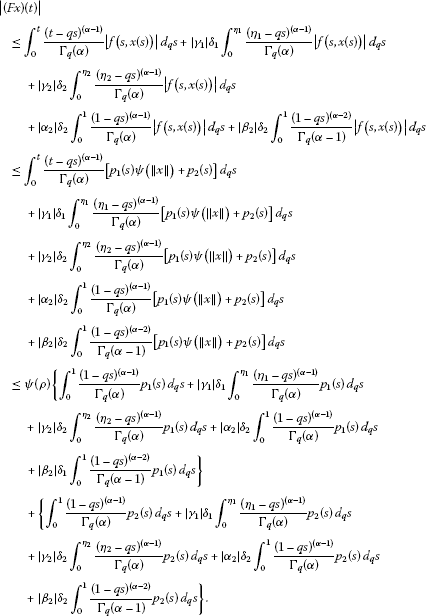Thus, for any $x\in {B}_{\rho }$ it holds

$\parallel Fx\parallel \le \psi \left(\rho \right){\omega }_{1}+{\omega }_{2},$

which proves our assertion.

Now we show that F maps bounded sets into equicontinuous sets of $C\left(\left[0,1\right],\mathbb{R}\right)$. Let ${t}_{1},{t}_{2}\in \left[0,1\right]$ with ${t}_{1}<{t}_{2}$ and $x\in {B}_{\rho }$, where ${B}_{\rho }$ is a bounded set of $C\left(\left[0,1\right],\mathbb{R}\right)$. Then taking into consideration the inequality ${\left({t}_{2}-qs\right)}^{\left(\alpha -1\right)}-{\left({t}_{1}-qs\right)}^{\left(\alpha -1\right)}\le \left({t}_{2}-{t}_{1}\right)$ for $0<{t}_{1}<{t}_{2}$ (see, , p.4]), we have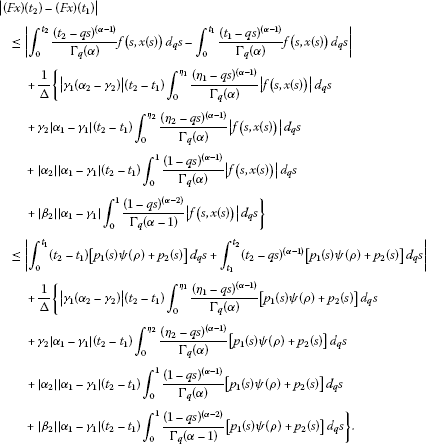Obviously, the right-hand side of the above inequality tends to zero independently of $x\in {B}_{\rho }$ as ${t}_{2}-{t}_{1}\to 0$. Therefore, it follows by the Arzelá-Ascoli theorem that $F:C\left(\left[0,1\right],\mathbb{R}\right)\to C\left(\left[0,1\right],\mathbb{R}\right)$ is completely continuous.

Thus, the operator F satisfies all the conditions of Lemma 3.6, and hence by its conclusion, either condition (i) or condition (ii) holds. We show that the conclusion (ii) is not possible.

Let $U=\left\{x\in C\left(\left[0,1\right],\mathbb{R}\right):\parallel x\parallel with $\psi \left(M\right){\omega }_{1}+{\omega }_{2} (by (3.8)). Then it can be shown that $\parallel Fx\parallel . Indeed, in view of (A4), we have

$\begin{array}{rcl}\parallel Fx\parallel & \le & \psi \left(\parallel x\parallel \right)\left\{{\int }_{0}^{1}\frac{{\left(1-qs\right)}^{\left(\alpha -1\right)}}{{\mathrm{\Gamma }}_{q}\left(\alpha \right)}{p}_{1}\left(s\right)\phantom{\rule{0.2em}{0ex}}{d}_{q}s+|{\gamma }_{1}|{\delta }_{1}{\int }_{0}^{{\eta }_{1}}\frac{{\left({\eta }_{1}-qs\right)}^{\left(\alpha -1\right)}}{{\mathrm{\Gamma }}_{q}\left(\alpha \right)}{p}_{1}\left(s\right)\phantom{\rule{0.2em}{0ex}}{d}_{q}s\\ +|{\gamma }_{2}|{\delta }_{2}{\int }_{0}^{{\eta }_{2}}\frac{{\left({\eta }_{2}-qs\right)}^{\left(\alpha -1\right)}}{{\mathrm{\Gamma }}_{q}\left(\alpha \right)}{p}_{1}\left(s\right)\phantom{\rule{0.2em}{0ex}}{d}_{q}s+|{\alpha }_{2}|{\delta }_{2}{\int }_{0}^{1}\frac{{\left(1-qs\right)}^{\left(\alpha -1\right)}}{{\mathrm{\Gamma }}_{q}\left(\alpha \right)}{p}_{1}\left(s\right)\phantom{\rule{0.2em}{0ex}}{d}_{q}s\\ +|{\beta }_{2}|{\delta }_{2}{\int }_{0}^{1}\frac{{\left(1-qs\right)}^{\left(\alpha -2\right)}}{{\mathrm{\Gamma }}_{q}\left(\alpha -1\right)}{p}_{1}\left(s\right)\phantom{\rule{0.2em}{0ex}}{d}_{q}s\right\}\\ +\left\{{\int }_{0}^{1}\frac{{\left(1-qs\right)}^{\left(\alpha -1\right)}}{{\mathrm{\Gamma }}_{q}\left(\alpha \right)}{p}_{2}\left(s\right)\phantom{\rule{0.2em}{0ex}}{d}_{q}s+|{\gamma }_{1}|{\delta }_{1}{\int }_{0}^{{\eta }_{1}}\frac{{\left({\eta }_{1}-qs\right)}^{\left(\alpha -1\right)}}{{\mathrm{\Gamma }}_{q}\left(\alpha \right)}{p}_{2}\left(s\right)\phantom{\rule{0.2em}{0ex}}{d}_{q}s\\ +|{\gamma }_{2}|{\delta }_{2}{\int }_{0}^{{\eta }_{2}}\frac{{\left({\eta }_{2}-qs\right)}^{\left(\alpha -1\right)}}{{\mathrm{\Gamma }}_{q}\left(\alpha \right)}{p}_{2}\left(s\right)\phantom{\rule{0.2em}{0ex}}{d}_{q}s+|{\alpha }_{2}|{\delta }_{2}{\int }_{0}^{1}\frac{{\left(1-qs\right)}^{\left(\alpha -1\right)}}{{\mathrm{\Gamma }}_{q}\left(\alpha \right)}{p}_{2}\left(s\right)\phantom{\rule{0.2em}{0ex}}{d}_{q}s\\ +|{\beta }_{2}|{\delta }_{2}{\int }_{0}^{1}\frac{{\left(1-qs\right)}^{\left(\alpha -2\right)}}{{\mathrm{\Gamma }}_{q}\left(\alpha -1\right)}{p}_{2}\left(s\right)\phantom{\rule{0.2em}{0ex}}{d}_{q}s\right\}\\ \le & \psi \left(M\right){\omega }_{1}+{\omega }_{2}

Suppose there exists a $x\in \partial U$ and a $\lambda \in \left(0,1\right)$ such that $x=\lambda Fx$. Then for such a choice of x and λ, we have

$M=\parallel x\parallel =\lambda \parallel Fx\parallel <\psi \left(\parallel x\parallel \right){\omega }_{1}+{\omega }_{2}=\psi \left(M\right){\omega }_{1}+{\omega }_{2}

which is a contradiction. Consequently, by the Leray-Schauder alternative (Lemma 3.6), we deduce that F has a fixed point $x\in \overline{U}$ which is a solution of the problem (1.1)-(1.2). This completes the proof. □

Remark 3.8 If ${p}_{1}$, ${p}_{2}$ in (A4) are continuous, then ${\omega }_{i}\le A\parallel {p}_{i}\parallel$, $i=1,2$, where A is defined by (3.5).

Example 3.9 Consider the fractional q-difference nonlocal boundary value problem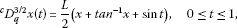(3.9)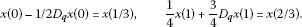(3.10)

Here, ${\alpha }_{1}=1$, ${\beta }_{1}=1/2$, ${\alpha }_{2}=1/4$, ${\beta }_{2}=3/4$, ${\gamma }_{1}=1={\gamma }_{2}$, ${\eta }_{1}=1/3$, ${\eta }_{2}=2/3$, and L is a constant to be fixed later on. Moreover, $\mathrm{\Delta }=5/8$, ${\delta }_{1}=26/15$, ${\delta }_{2}=4/3$, $|f\left(t,x\right)-f\left(t,y\right)|\le L|x-y|$, and

$k=\frac{L}{{\mathrm{\Gamma }}_{1/2}\left(3/2\right)}\left(\frac{2\sqrt{2}\left(13+10\sqrt{2}+15\sqrt{3}\right)}{15\sqrt{3}\left(2\sqrt{2}-1\right)}+1\right).$

Choosing

$L<{\left[\frac{1}{{\mathrm{\Gamma }}_{1/2}\left(3/2\right)}\left(\frac{2\sqrt{2}\left(13+10\sqrt{2}+15\sqrt{3}\right)}{15\sqrt{3}\left(2\sqrt{2}-1\right)}+1\right)\right]}^{-1},$

all the assumptions of Theorem 3.1 are satisfied. Therefore, by Theorem 3.1, problem (3.9)-(3.10) has a unique solution.

Example 3.10 Consider the problem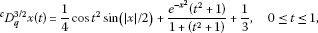(3.11)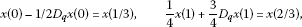(3.12)

where ${\alpha }_{1}=1$, ${\beta }_{1}=1/2$, ${\alpha }_{2}=1/4$, ${\beta }_{2}=3/4$, ${\gamma }_{1}=1={\gamma }_{2}$, ${\eta }_{1}=1/3$, ${\eta }_{2}=2/3$. In a straightforward manner, it can be found that $\mathrm{\Delta }=5/8$, ${\delta }_{1}=26/15$, f${\delta }_{2}=4/3$, and

$|f\left(t,x\right)|=|\frac{1}{4}cos{t}^{2}sin\left(|x|/2\right)+\frac{{e}^{-{x}^{2}}\left({t}^{2}+1\right)}{1+\left({t}^{2}+1\right)}+\frac{1}{3}|\le \frac{1}{8}|x|+1.$

Clearly, ${p}_{1}=1/8$, ${p}_{2}=1$, $\psi \left(M\right)=M$. Consequently, ${\omega }_{1}=0.567120414$, ${\omega }_{2}=4.536963312$, and the condition (3.8) implies that $M>10.48055997$. Thus, all the assumptions of Theorem 3.7 are satisfied. Therefore, the conclusion of Theorem 3.7 applies to the problem (3.11)-(3.12).

## References

1. Podlubny I: Fractional Differential Equations. Academic Press, San Diego; 1999.

2. Kilbas AA, Srivastava HM, Trujillo JJ North-Holland Mathematics Studies 204. In Theory and Applications of Fractional Differential Equations. Elsevier, Amsterdam; 2006.

3. Sabatier J, Agrawal OP, Machado JAT (Eds): Advances in Fractional Calculus: Theoretical Developments and Applications in Physics and Engineering. Springer, Dordrecht; 2007.

4. Baleanu D, Diethelm K, Scalas E, Trujillo JJ Series on Complexity, Nonlinearity and Chaos. In Fractional Calculus Models and Numerical Methods. World Scientific, Boston; 2012.

5. Agarwal RP, Belmekki M, Benchohra M: A survey on semilinear differential equations and inclusions involving Riemann-Liouville fractional derivative. Adv. Differ. Equ. 2009., 2009: Article ID 981728

6. Gafiychuk V, Datsko B, Meleshko V: Mathematical modeling of different types of instabilities in time fractional reaction-diffusion systems. Comput. Math. Appl. 2010, 59: 1101–1107. 10.1016/j.camwa.2009.05.013

7. Ahmad B, Agarwal RP: On nonlocal fractional boundary value problems. Dyn. Contin. Discrete Impuls. Syst. 2011, 18: 535–544.

8. Baleanu D, Mustafa OG, O’Regan D: A Nagumo-like uniqueness theorem for fractional differential equations. J. Phys. A, Math. Theor. 2011., 44(39): Article ID 392003

9. Wang Y, Liu L, Wu Y: Positive solutions for a nonlocal fractional differential equation. Nonlinear Anal. 2011, 74: 3599–3605. 10.1016/j.na.2011.02.043

10. Ford NJ, Morgado ML: Fractional boundary value problems: analysis and numerical methods. Fract. Calc. Appl. Anal. 2011, 14: 554–567.

11. Ahmad B, Nieto JJ, Alsaedi A, El-Shahed M: A study of nonlinear Langevin equation involving two fractional orders in different intervals. Nonlinear Anal., Real World Appl. 2012, 13: 599–606. 10.1016/j.nonrwa.2011.07.052

12. Ahmad B, Ntouyas SK: Nonlinear fractional differential equations and inclusions of arbitrary order and multi-strip boundary conditions. Electron. J. Differ. Equ. 2012, 2012(98):1–22.

13. Chen F, Nieto JJ, Zhou Y: Global attractivity for nonlinear fractional differential equations. Nonlinear Anal., Real World Appl. 2012, 13: 287–298. 10.1016/j.nonrwa.2011.07.034

14. Jackson FH: On q -difference equations. Am. J. Math. 1910, 32: 305–314. 10.2307/2370183

15. Jackson FH: On qq-functions and a certain difference operator. Trans. R. Soc. Edinb. 1908, 46: 253–281.

16. Ernst, T: A new notation for q-calculus and a new q-Taylor formula. U.U.D.M. Report 1999:25, Department of Mathematics, Uppsala University (1999). ISSN:1101–3591

17. Ernst, T: The history of q-calculus and a new method. U.U.D.M. Report 2000:16, Department of Mathematics, Uppsala University (2000). ISSN:1101–3591

18. Ahmad B: Boundary-value problems for nonlinear third-order q -difference equations. Electron. J. Differ. Equ. 2011, 2011(94):1–7.

19. Ahmad B, Ntouyas SK: Boundary value problems for q -difference inclusions. Abstr. Appl. Anal. 2011., 2011: Article ID 292860

20. Ahmad B, Alsaedi A, Ntouyas SK: A study of second-order q -difference equations with boundary conditions. Adv. Differ. Equ. 2012., 2012: Article ID 35

21. Atici FM, Eloe PW: A transform method in discrete fractional calculus. Int. J. Differ. Equ. 2007, 2: 165–176.

22. Goodrich CS: Solutions to a discrete right-focal fractional boundary value problem. Int. J. Differ. Equ. 2010, 5: 195–216.

23. Goodrich CS: Continuity of solutions to discrete fractional initial value problems. Comput. Math. Appl. 2010, 59: 3489–3499. 10.1016/j.camwa.2010.03.040

24. Atici FM, Eloe PW: Linear systems of fractional nabla difference equation. Rocky Mt. J. Math. 2011, 41: 353–360. 10.1216/RMJ-2011-41-2-353

25. Atici FM, Eloe PW: Two-point boundary value problems for finite fractional difference equations. J. Differ. Equ. Appl. 2011, 17: 445–456. 10.1080/10236190903029241

26. Bastos NRO, Ferreira RAC, Torres DFM: Discrete-time fractional variational problems. Signal Process. 2011, 91: 513–524. 10.1016/j.sigpro.2010.05.001

27. Bastos NRO, Mozyrska D, Torres DFM: Fractional derivatives and integrals on time scales via the inverse generalized Laplace transform. Int. J. Math. Comput. 2011, 11: 1–9.

28. Goodrich CS: Existence of a positive solution to a system of discrete fractional boundary value problems. Appl. Math. Comput. 2011, 217: 4740–4753. 10.1016/j.amc.2010.11.029

29. Goodrich CS: On discrete sequential fractional boundary value problems. J. Math. Anal. Appl. 2012, 385: 111–124. 10.1016/j.jmaa.2011.06.022

30. Al-Salam WA: Some fractional q -integrals and q -derivatives. Proc. Edinb. Math. Soc. 1966–1967, 15: 135–140. 10.1017/S0013091500011469

31. Agarwal R: Certain fractional q -integrals and q -derivatives. Proc. Camb. Philos. Soc. 1969, 66: 365–370. 10.1017/S0305004100045060

32. El-Shahed M, Al-Askar F: Positive solutions for boundary value problem of nonlinear fractional q -difference equation. ISRN Math. Anal. 2011., 2011: Article ID 385459

33. Ferreira RAC: Positive solutions for a class of boundary value problems with fractional q -differences. Comput. Math. Appl. 2011, 61: 367–373. 10.1016/j.camwa.2010.11.012

34. Ferreira RAC: Nontrivial solutions for fractional q -difference boundary value problems. Electron. J. Qual. Theory Differ. Equ. 2010, 2010(70):1–10.

35. Graef JR, Kong L: Positive solutions for a class of higher order boundary value problems with fractional q -derivatives. Appl. Math. Comput. 2012. doi:10.1016/j.amc.2012.03.006

36. Ma J, Yang J: Existence of solutions for multi-point boundary value problem of fractional q -difference equation. Electron. J. Qual. Theory Differ. Equ. 2011, 2011(92):1–10.

37. Gasper G, Rahman M: Basic Hypergeometric Series. Cambridge University Press, Cambridge; 1990.

38. Kac V, Cheung P: Quantum Calculus. Springer, New York; 2002.

39. Rajkovic PM, Marinkovic SD, Stankovic MS: On q -analogues of Caputo derivative and Mittag-Leffler function. Fract. Calc. Appl. Anal. 2007, 10: 359–373.

40. Rajkovic PM, Marinkovic SD, Stankovic MS: Fractional integrals and derivatives in q -calculus. Appl. Anal. Discrete Math. 2007, 1: 311–323. 10.2298/AADM0701311R

41. Smart DR: Fixed Point Theorems. Cambridge University Press, Cambridge; 1980.

42. Granas A, Dugundji J: Fixed Point Theory. Springer, New York; 2005.

## Acknowledgements

The research of B. Ahmad was partially supported by Deanship of Scientific Research (DSR), King Abdulaziz University, Jeddah, Saudi Arabia. The authors thank the reviewers for their useful comments.

## Author information

Authors

### Corresponding author

Correspondence to Sotiris K Ntouyas.

### Competing interests

The authors declare that they have no competing interests.

### Authors’ contributions

Each of the authors, BA, SKN, and IKP contributed to each part of this study equally and read and approved the final version of the manuscript.

## Rights and permissions

Reprints and Permissions

Ahmad, B., Ntouyas, S.K. & Purnaras, I.K. Existence results for nonlocal boundary value problems of nonlinear fractional q-difference equations. Adv Differ Equ 2012, 140 (2012). https://doi.org/10.1186/1687-1847-2012-140

• Accepted:

• Published:

• DOI: https://doi.org/10.1186/1687-1847-2012-140

### Keywords

• fractional q-difference equations
• nonlocal boundary conditions
• existence
• Leray-Schauder nonlinear alternative
• fixed point